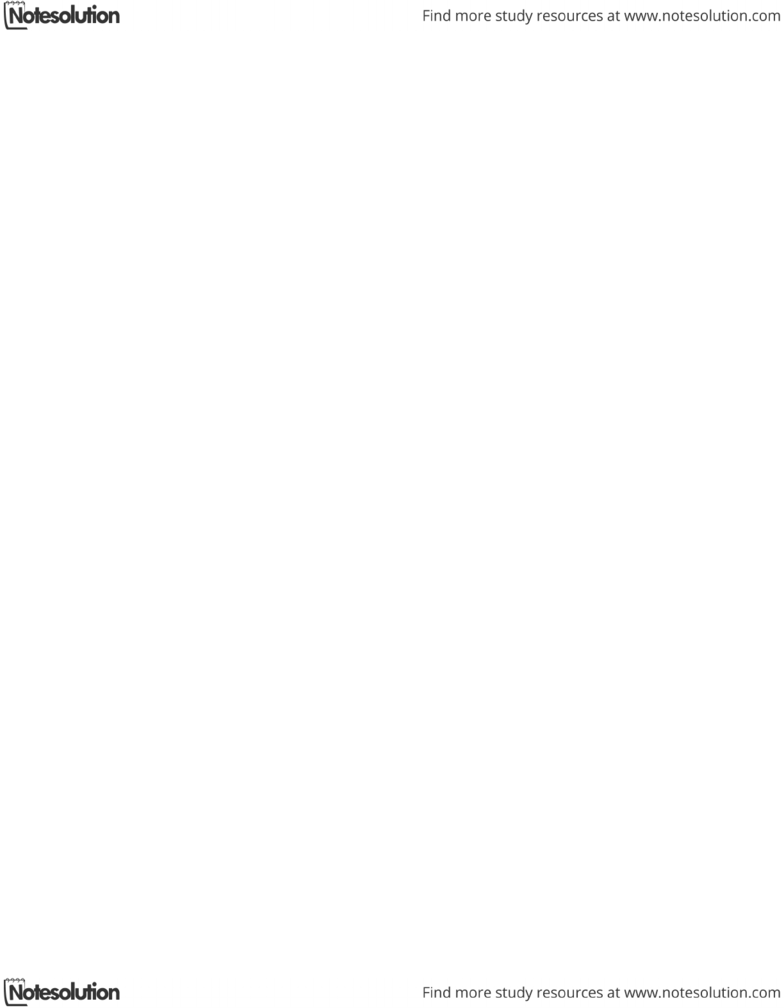Textbook Notes (290,000)
CA (170,000)
Western (10,000)
2241A/B (10)
Chapter

# Kinesiology 2241A/B Chapter Notes -Buoyancy

Department
Kinesiology
Course Code
Kinesiology 2241A/B
Professor
Bob Vigars

Page:
of 2Concept Module E Torque and Rotation
When a force is applied to an object, it moves the object’s C of G in a linear
path
When the object is restricted to movement about an axis, the force causes the
object to rotate
o Except when the force is applied directly at the axis of rotation
Eccentric force off-center force that causes torque
Force arm the off-axis distance to the force’s line of action
o The greater distance, the greater torque produced by the force
o The shortest distance from the axis of rotation to the line of action of
the force
o Always the length of the line that is perpendicular to the force’s line of
action
Four properties of torque-producing forces:
o Magnitude
o Direction
o Line of action
o Point of application
Torque is produced by muscles when they pull on bones, and the result is
rotary motion of the body segments about an axis
The stronger the muscle contraction, the greater torque on the bone
The longer the force arm, the greater the torque
Teeter-totter:
o If the weight is less (ie. a child), it creates less torque
o If the weight is placed closer to the axis it creates less torque
If many torques are applied to a system, the sum of the torques tells the
movement direction (net torque)
The vectors representing the direction of the torques on a system can be
determined using the right hand thumb rule
Clockwise is negative, counterclockwise is positive
When an object is free to travel, both linear and rotary motion can occur
The force of gravity on an object is equal to its weight
The C of G is towards the more massive ends of objects wherever the body
parts balance
For a system to be in balance, the opposing torques on each side of the
fulcrum must be equal
o The sum of the torques on one side is equal and opposite to the sum of
the opposing torques on the other side
o If the system is balanced, the fulcrum is in vertical alignment with the
C of G of the system
As the body moves, the C of G moves so there is always an equal distribution
of mass on all sides of the point
Center of Buoyancy (C of B) the point that is the C of G of a volume of water
equal to the volume and shape of the submerged body
Generally, the C of B is more in the chest region and the C of G is closer to the
pelvic region
When the C of B and the C of G are in different locations in the body, a torque
will be created and the body will rotate
o The further they are apart, the more the body will rotate (greater
torque)
When the C of B and the C of G are collinear, there will be no torque/rotation
(above or below each other, but in a vertical line so the forces cancel out)